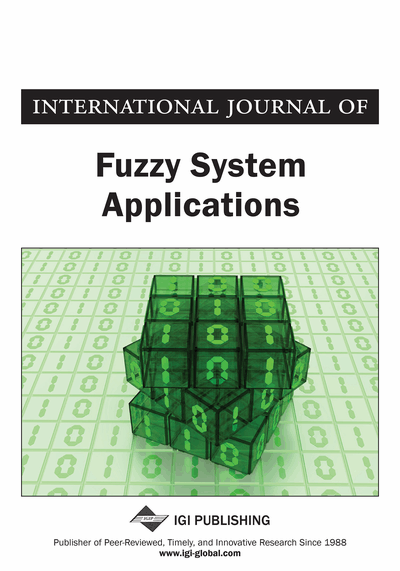# On the Latest Times and Float Times of Activities in a Fuzzy Project Network with LR Fuzzy Numbers

V. Sireesha (GITAM University, India), N. Ravi Shankar (GITAM University, India), K. Srinivasa Rao (GITAM University, India), and P. Phani Bushan Rao (GITAM University, India)
DOI: 10.4018/ijfsa.2012040105
Available
\$29.50
No Current Special Offers

## Abstract

In this paper, the authors propose a new method to compute the fuzzy latest times and float times of activities for a project scheduling problem with fuzzy activity times. The authors have considered LR fuzzy numbers to represent the activity times. As the data of the problem are LR fuzzy numbers, the authors have shown that the results are also in terms of LR fuzzy numbers. Total float time of each activity can be found by this method without using the forward pass and backward pass computations. The authors use an example to illustrate the method. This paper shows the advantages of this method over the existing methods with great clarity. The proposed method illustrates its application to fuzzy critical path problems occurring in real life situations.
Article Preview
Top

## Introduction

The critical path method (CPM) (Kelley, 1961) is a network based method useful in practice and control of complex projects. The activity durations in the CPM are deterministic and known, although precise information about the durations of activities is seldom available. To deal quantitatively with imprecise durations, the program evaluation and review technique (PERT) (Malcolm, 1959) and Monte Carlo simulation (Ragsdale, 1989; Kurihara et al., 2002) based on the probability theory can be employed. In PERT, the exact determination of the total project duration is generally intractable, except when the graph is a series-parallel one (Dubois et al., 2003). Thus, approximation techniques, like transforming the original network into a series-parallel one (Dobin, 1985), have been proposed. Due to the uniqueness of projects, historical data about activity durations are not available. As activity durations have to be estimated by human experts, under unique circumstances, project management is faced with judgmental statements that are imprecise. Among the three principle aspects of project management, viz. time, uncertainty, and simulation, the handling of uncertainties plays a major role. In real applications there is a great need for handling situations on a higher uncertainty level. Such situations occur in connection with unique, innovative, and costly projects and tests, or start-ups of systems of a new type. In those situations, rather than random variables (Herroelen, 2005), Shipley et al. (1997), and Lootsma (1989) have compared the fuzzy approach with the PERT approach.

Chanas and colleagues proposed a series studies on the topic of the fuzzy project scheduling. For example, Chanas et al. (2002) studied necessarily critical activities; Chanas and Zielinski (2002, 2003) discussed the complexity of criticality; Chanas and Zielinski (2001) proposed two methods of calculating the degree of possible criticality of paths. Problems related to necessarily and possibly critical paths in networks with imprecise activity and time lag durations have been discussed by Yakhchali et al. (2008), Chen and Hsueh (2008), and Chen (2007) proposed an approach based on the extension principle and linear programming formulation to critical path analysis in networks with fuzzy activity durations. Chen and Huang (2007) combined fuzzy set theory with the traditional methods to compute the critical degrees of activities and paths. Yakhchali et al. (2008) discussed project scheduling problem with irregular starting time costs in networks with imprecise durations. Fuzzy scheduling is not only applied for project scheduling but also in various scheduling problems like fabric-cutting scheduling (Kwong et al., 2006; Mok et al., 2007). Rong et al. (2008) considered uncertainty related the lead-time and developed a single wholesaler and multi retailers mixture inventory distribution model. Gue et al. (2008) considered uncertain processing time, orders and arrival time and proposed a genetic algorithm for an order scheduling problem at the factory level. Kumar and Kaur (2010) proposed a new method to find the fuzzy optimal solution of fully fuzzy critical path problems. Sireesha and Shankar (2010) presented a new method based on fuzzy theory for solving fuzzy project scheduling in fuzzy environment. Assuming that the duration of activities are triangular fuzzy numbers, they computed total float time of each activity and fuzzy critical path without computing forward and backward pass calculations. Kumar and Kaur (2011) proposed a new method that modifies the existing one. They presented the advantages of the proposed method by solving a specific fuzzy critical path problem. Shankar et al. (2010) presented an analytical method for measuring the criticality in a fuzzy project network, where the duration time of each activity is represented by a trapezoidal fuzzy number. They used a new defuzzification formula for trapezoidal fuzzy number and apply to the float time for each activity in the fuzzy project network to find fuzzy critical path.

## Complete Article List

Search this Journal:
Reset
Volume 12: 1 Issue (2023)
Volume 11: 4 Issues (2022)
Volume 10: 4 Issues (2021)
Volume 9: 4 Issues (2020)
Volume 8: 4 Issues (2019)
Volume 7: 4 Issues (2018)
Volume 6: 4 Issues (2017)
Volume 5: 4 Issues (2016)
Volume 4: 4 Issues (2015)
Volume 3: 4 Issues (2013)
Volume 2: 4 Issues (2012)
Volume 1: 4 Issues (2011)
View Complete Journal Contents Listing